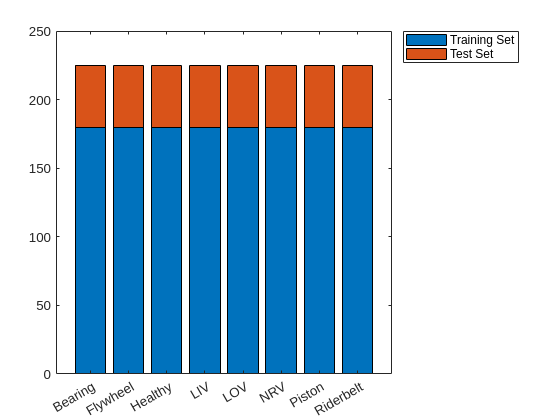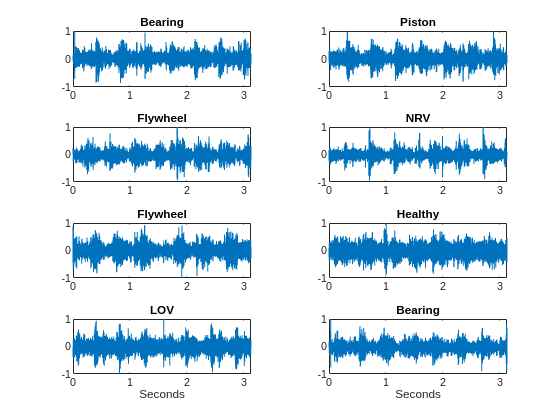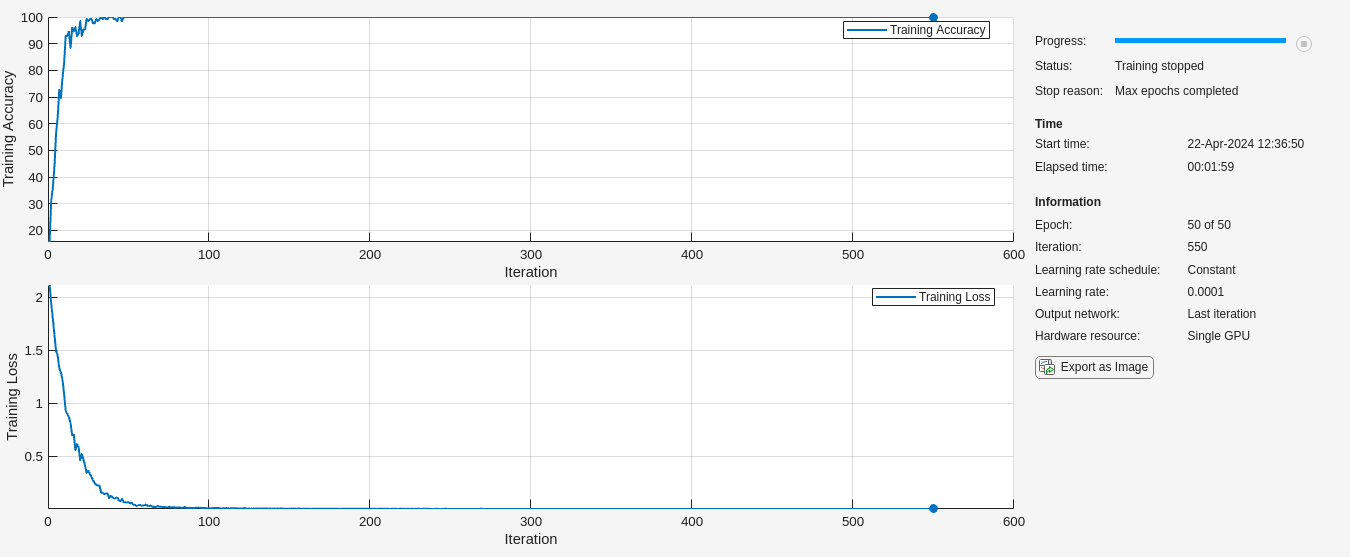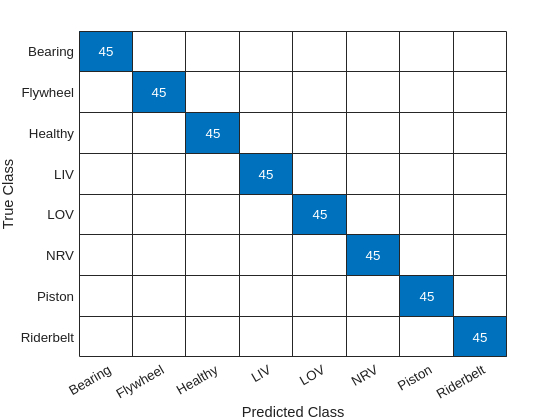# Fault Detection Using Wavelet Scattering and Recurrent Deep Networks

This example shows how to classify faults in acoustic recordings of air compressors using a wavelet scattering network paired with a recurrent neural network. The example provides the opportunity to use a GPU to accelerate the computation of the wavelet scattering transform. If you wish to utilize a GPU, you must have Parallel Computing Toolbox™ and a supported GPU. See GPU Computing Requirements (Parallel Computing Toolbox) for details.

### Dataset

The dataset consists of acoustic recordings collected on a single stage reciprocating type air compressor . The data are sampled at 16 kHz. Specifications of the air compressor are as follows:

• Air Pressure Range: 0-500 lb/m2, 0-35 Kg/cm2

• Induction Motor: 5HP, 415V, 5Am, 50 Hz, 1440rpm

• Pressure Switch: Type PR-15, Range 100-213 PSI

Each recording represents one of 8 states which includes the healthy state and 7 faulty states. The 7 faulty states are:

1. Leakage inlet valve (LIV) fault

2. Leakage outlet valve (LOV) fault

3. Non-return valve (NRV) fault

4. Piston ring fault

5. Flywheel fault

6. Rider belt fault

7. Bearing fault

Download the dataset and unzip the data file in a folder where you have write permission. This example assumes you are downloading the data in the temporary directory designated as `tempdir` in MATLAB®. If you chose to use a different folder, substitute that folder for `tempdir` in the following. The recordings are stored as .wav files in folders named for their respective state.

```url = 'https://www.mathworks.com/supportfiles/audio/AirCompressorDataset/AirCompressorDataset.zip'; downloadFolder = fullfile(tempdir,'AirCompressorDataSet'); if ~exist(fullfile(tempdir,'AirCompressorDataSet'),'dir') loc = websave(downloadFolder,url); unzip(loc,fullfile(tempdir,'AirCompressorDataSet')) end```

Use an `audioDatastore` to manage data access. Each subfolder contains only recordings of the designated class. Use the folder names as the class labels.

```datasetLocation = fullfile(tempdir,'AirCompressorDataSet','AirCompressorDataset'); ads = audioDatastore(datasetLocation,'IncludeSubfolders',true,... 'LabelSource','foldernames');```

Examine the number of examples in each class. There are 225 recordings in each class.

`countcats(ads.Labels)`
```ans = 8×1 225 225 225 225 225 225 225 225 ```

Split the data into training and test sets. Use 80% of the data for training and hold out the remaining 20% for testing. Shuffle the data once before splitting.

```rng default ads = shuffle(ads); [adsTrain,adsTest] = splitEachLabel(ads,0.8,0.2);```

Verify that the number of examples in each class is the expected number.

```uniqueLabels = unique(adsTrain.Labels); tblTrain = countEachLabel(adsTrain); tblTest = countEachLabel(adsTest); H = bar(uniqueLabels,[tblTrain.Count, tblTest.Count],'stacked'); legend(H,["Training Set","Test Set"],'Location','NorthEastOutside')```Select some random examples from the training set for plotting.

```idx = randperm(numel(adsTrain.Files),8); Fs = 16e3; for n = 1:numel(idx) x = audioread(adsTrain.Files{idx(n)}); t = (0:size(x,1)-1)/Fs; subplot(4,2,n); plot(t,x); if n == 7 || n == 8 xlabel('Seconds'); end title(string(adsTrain.Labels(idx(n)))); end```### Wavelet Scattering Network

Each record has 50,000 samples sampled at 16 kHz. Construct a wavelet scattering network based on the data characteristics. Set the invariance scale to be 0.5 seconds.

```N = 5e4; Fs = 16e3; IS = 0.5; sn = waveletScattering('SignalLength',N,'SamplingFrequency',Fs,... 'InvarianceScale',0.5);```

With these network settings, there are 330 scattering paths and 25 time windows per example. You can see this with the following code.

```[~,npaths] = paths(sn); Ncfs = numCoefficients(sn); sum(npaths)```
```ans = 330 ```
`Ncfs`
```Ncfs = 25 ```

Note this already represents a 6-fold reduction in the size of the data for each record. We reduced the data size from 50,000 samples to 8250 in total. Most importantly, we reduced the size of the data along the time dimension from 50,000 to 25 samples. This is crucial for our use of a recurrent network. Attempting to use a recurrent network on the original data with 50,000 samples would immediately result in memory problems.

Obtain the wavelet scattering features for the training and test sets. If you have a suitable GPU and Parallel Computing Toolbox, you can set `useGPU` to `true` to accelerate the scattering transform. The function `helperBatchScatFeatures` obtains the scattering transform of each example.

```batchsize = 64; useGPU = false; scTrain = []; while hasdata(adsTrain) sc = helperBatchScatFeatures(adsTrain,sn,N,batchsize,useGPU); scTrain = cat(3,scTrain,sc); end```

Repeat the process for the held out test set.

```scTest = []; while hasdata(adsTest) sc = helperBatchScatFeatures(adsTest,sn,N,batchsize,useGPU); scTest = cat(3,scTest,sc); end```

Remove the 0-th order scattering coefficients. For both the training and test sets, put each 330-by-25 scattering transform into an element of a cell array for use in training and testing the recurrent network.

```TrainFeatures = scTrain(2:end,:,:); TrainFeatures = squeeze(num2cell(TrainFeatures,[1 2])); YTrain = adsTrain.Labels; TestFeatures = scTest(2:end,:,:); TestFeatures = squeeze(num2cell(TestFeatures,[1 2])); YTest = adsTest.Labels;```

### Define Network

Recall there are 1440 training examples and 360 test set examples. Accordingly the `TrainFeatures` and `TestFeatures` cell arrays have 1440 and 360 elements respectively.

Use the number of scattering paths as the number of features. Create a recurrent network with a single LSTM layer having 512 hidden units. Follow the LSTM layer with a fully connected layer and finally a softmax layer. Use 'zscore' normalization across all scattering paths at the input to the network.

```[inputSize, ~] = size(TrainFeatures{1}); numHiddenUnits = 512; numClasses = numel(unique(YTrain)); layers = [ ... sequenceInputLayer(inputSize,'Normalization','zscore') lstmLayer(numHiddenUnits,'OutputMode','last') fullyConnectedLayer(numClasses) softmaxLayer classificationLayer];```

### Train Network

Train the network for 50 epochs with a mini batch size of 128. Use an Adam optimizer with an initial learn rate of 1e-4. Shuffle the data each epoch.

```maxEpochs = 50; miniBatchSize = 128; options = trainingOptions('adam', ... 'InitialLearnRate',1e-4,... 'MaxEpochs',maxEpochs, ... 'MiniBatchSize',miniBatchSize, ... 'SequenceLength','shortest', ... 'Shuffle','every-epoch',... 'Plots','training-progress',... 'Verbose',true); net = trainNetwork(TrainFeatures,YTrain,layers,options);```
```Training on single CPU. Initializing input data normalization. |========================================================================================| | Epoch | Iteration | Time Elapsed | Mini-batch | Mini-batch | Base Learning | | | | (hh:mm:ss) | Accuracy | Loss | Rate | |========================================================================================| | 1 | 1 | 00:00:08 | 16.41% | 2.1281 | 1.0000e-04 | | 5 | 50 | 00:00:59 | 100.00% | 0.0536 | 1.0000e-04 | | 10 | 100 | 00:01:51 | 100.00% | 0.0072 | 1.0000e-04 | | 14 | 150 | 00:02:40 | 100.00% | 0.0064 | 1.0000e-04 | | 19 | 200 | 00:03:27 | 100.00% | 0.0025 | 1.0000e-04 | | 23 | 250 | 00:04:14 | 100.00% | 0.0015 | 1.0000e-04 | | 28 | 300 | 00:05:00 | 100.00% | 0.0012 | 1.0000e-04 | | 32 | 350 | 00:05:45 | 100.00% | 0.0007 | 1.0000e-04 | | 37 | 400 | 00:06:29 | 100.00% | 0.0006 | 1.0000e-04 | | 41 | 450 | 00:07:12 | 100.00% | 0.0005 | 1.0000e-04 | | 46 | 500 | 00:07:55 | 100.00% | 0.0005 | 1.0000e-04 | | 50 | 550 | 00:08:41 | 100.00% | 0.0004 | 1.0000e-04 | |========================================================================================| Training finished: Reached final iteration. ```In training, the network has achieved near perfect performance. In order to ensure we have not overfit to the training data, use the held-out test set to determine how well our network generalizes to unseen data.

```YPred = classify(net,TestFeatures); accuracy = 100*sum(YPred == YTest) / numel(YTest)```
```accuracy = 100 ```

In this case, we see that the performance on the held-out test set is also excellent.

```figure confusionchart(YTest, YPred)```### Summary

In this example, the wavelet scattering transform was used with a simple recurrent network to classify faults in an air compressor. The scattering transform allowed us to extract robust features for our learning problem. Additionally, the data reduction achieved along the time dimension of the data by the use of the wavelet scattering transform was critical in order to create a computationally feasible problem for our recurrent network.

### References

 Verma, Nishchal K., Rahul Kumar Sevakula, Sonal Dixit, and Al Salour. “Intelligent Condition Based Monitoring Using Acoustic Signals for Air Compressors.” IEEE Transactions on Reliability 65, no. 1 (March 2016): 291–309. https://doi.org/10.1109/TR.2015.2459684.

helperbatchscatfeatures - This function returns the wavelet time scattering feature matrix for a given input signal. If `useGPU` is set to `true`, the scattering transform is computed on the GPU.

```function sc = helperBatchScatFeatures(ds,sn,N,batchsize,useGPU) % This function is only intended to support examples in the Wavelet % Toolbox. It may be changed or removed in a future release. % Read batch of data from audio datastore batch = helperReadBatch(ds,N,batchsize); if useGPU batch = gpuArray(batch); end % Obtain scattering features S = sn.featureMatrix(batch,'transform','log'); gather(batch); S = gather(S); % Subsample the features %sc = S(:,1:6:end,:); sc = S; end```

helperReadBatch - This function reads batches of a specified size from a datastore and returns the output in single precision. Each column of the output is a separate signal from the datastore. The output may have fewer columns than the batch size if the datastore does not have enough records.

```function batchout = helperReadBatch(ds,N,batchsize) % This function is only in support of Wavelet Toolbox examples. It may % change or be removed in a future release. % % batchout = readReadBatch(ds,N,batchsize) where ds is the Datastore and % ds is the Datastore % batchsize is the batchsize kk = 1; while(hasdata(ds)) && kk <= batchsize tmpRead = read(ds); batchout(:,kk) = cast(tmpRead(1:N),'single'); %#ok<AGROW> kk = kk+1; end end```